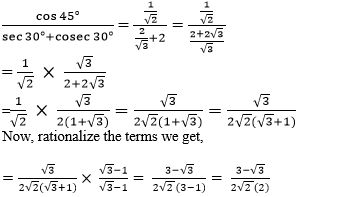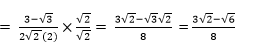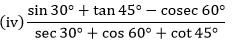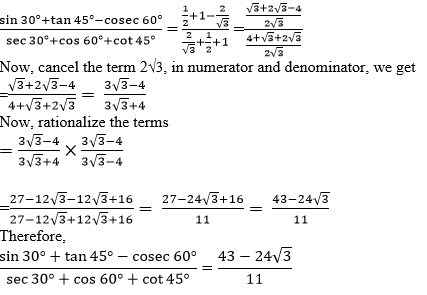# Chapter 8 – Introduction to Trigonometry Questions and Answers: NCERT Solutions for Class 10 Mathematics

Class 10 Mathematics NCERT book solutions for Chapter 8 - Introduction to Trigonometry Questions and Answers.

## 1. Evaluate the following: (i) sin 60° cos 30° + sin 30° cos 60° (ii) 2 tan2 45° + cos2 30° – sin2 60

###Now, multiply both the numerator and denominator by √2 , we getTherefore, cos 45°/(sec 30°+cosec 30°) = (3√2 – √6)/8

###We know that, sin 30° = 1/2 tan 45° = 1 cosec 60° = 2/√3 sec 30° = 2/√3 cos 60° = 1/2 cot 45° = 1 Substitute the values in the given problem, we get